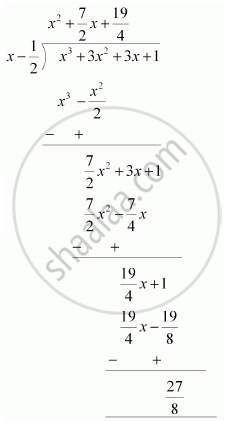# Find the remainder when x^3 + 3x^2 + 3x + 1 is divided by x - 1/2 - Mathematics

Find the remainder when x3 + 3x2 + 3x + 1 is divided by x - 1/2

#### Solution 1

Let p(x) = x3 + 3x2 + 3x + 1

x-1/2 = 0 ⇒ x = 1/2

therefore "Remainder "= (1/2)^3 + 3(1/2)^2 + 3(1/2) + 1

= 1/8 +3/4+3/2+1

= 27/8

Therefore, the remainder is 27/8" ."

#### Solution 2

By long division,Therefore, the remainder is 27/8" ."

Concept: Remainder Theorem
Is there an error in this question or solution?

#### APPEARS IN

NCERT Class 9 Maths
Chapter 2 Polynomials
Exercise 2.3 | Q 1.2 | Page 40